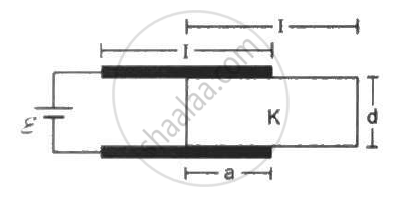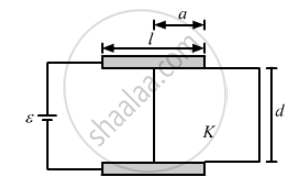Department of Pre-University Education, Karnataka course PUC Karnataka Science Class 12
Share

# Consider the Situation Shown in Figure . the Plates of the Capacitor Have Plate Area a and Are Clamped in the Laboratory. the Dielectric Slab is Released from Rest - Physics

ConceptCapacitors and Capacitance

#### Question

Consider the situation shown in figure. The plates of the capacitor have plate area A and are clamped in the laboratory. The dielectric slab is released from rest with a length a inside the capacitor. Neglecting any effect of friction or gravity, show that the slab will execute periodic motion and find its time period.#### SolutionGiven that the area of the plates of the capacitors is A.

As ''a'' length of the dielecric slab is inside the capacitor.

Therefore, the area of the plate covered with dielectric is = A/la

The capacitance of the portion with dielectric is given by C_1 = (K∈_0Aa)/(ld)

The capacitance of the portion without dielectric is given by C_1 = (∈_0A(l-a))/(ld)

The two parts can be considered to be in parallel.

Therefore, the net capacitance is given by

C = C_1 + C_2

⇒ C = (∈_0A)/(ld)[Ka + (l-a)]

⇒ C = (∈_0A)/(ld)[l + a(K-1)]

Let us consider a small displacement da of the slab in the inward direction. The capacitance will increase, therefore the energy of the capacitor will also increase. In order to maintain constant voltage, the battery will supply extra charges, therefore the battery will do work.

Work done by the battery = change in energy of capacitor + work done by the force F on the capacitor

dW_B = dU + dW_F

Let the charge dq is supplied by the battery, and the change in the capacitor be dC

dW_B = (dq).V = (dC).V^2

dU = 1/2(dC).V^2

(dC).V^2 = 1/2(dC).V^2 + F.da

1/2(dC).V^2 = F.da

⇒ F = 1/2 (dC)/(da)V^2

⇒ F = 1/2 d/(da) ((∈_0A)/(ld)[l + a(K-1)])V^2

⇒ F = 1/2 (∈_0A)/(ld) (K - 1)

The acceleration of the dielectric is given by a_0 = 1/2 (∈_0A)/(ldm)(K-1)

As, the force is in inward direction, it will tend to make the dielectric to completely fill the space inside the capacitors. As, the dielectric completely fills the space inside the capacitor at this instant its velocity is not zero. The dielectric slab tends to move outside the capacitor. As the slab tends to move out, the direction of the force due to the capacitor will reverse its direction. Thus, the dielectric slab will have a periodic motion.

The time taken to move distance (l-a) can be calculated as :-

(l - a) = 1/2 a_0t^2

t = sqrt ((2(l-a))/(a_0))

t = sqrt (2(l-a) xx (2ldm)/(∈_0AV^2(K-1))

t = sqrt ((4m(l-a)ld)/(∈_0AV^2(K-1))

For the complete cycle the time period will be four times the time taken for covering distance (l-a).

It is given by :-

T = 4t = 4 xx 2 sqrt((m(l-a)ld)/(∈_0AV^2(K-1))) = 8 sqrt ((m(l-a)ld)/(∈_0AV^2(K-1))

Is there an error in this question or solution?

#### Video TutorialsVIEW ALL 

Solution Consider the Situation Shown in Figure . the Plates of the Capacitor Have Plate Area a and Are Clamped in the Laboratory. the Dielectric Slab is Released from Rest Concept: Capacitors and Capacitance.
S